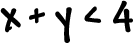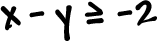Ok, now let's do a system: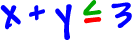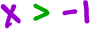We just graph each one and see where they overlap.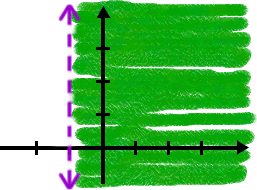What will the intersection look like?

 Knowing what this point is is going to be really important in the next section! It LOOKS like it's (-1, 4), but we need to be sure.  Remember that the point of intersection is the solution to the system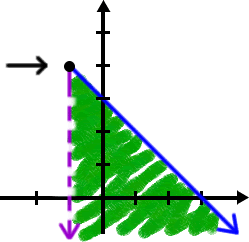We have the x guy, so stick him into the other equation:Yep, the point is (-1, 4), so here's our official answer:Graph